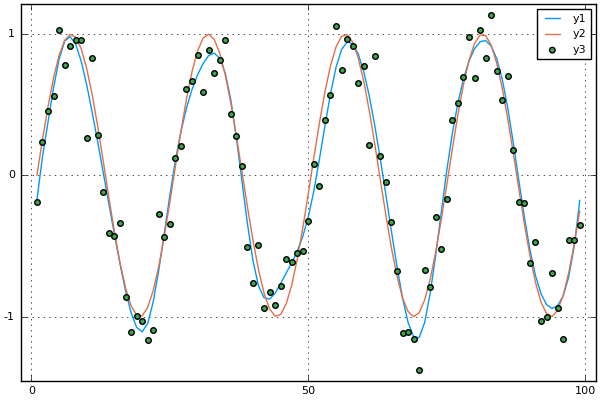# Regression splines tutorial

This tutorial describes the spline basis and smoothing techniques which are based on splines.

using Plots
pyplot()


Here is a simple function and noisy data. The object of this tutorial is to estimate the sinuosoidal curve given the noisy observations.

x = linspace(0, 2*pi, 100)[1:end-1]
y = sin(4*x)
yn = y + randn(size(y)) *.2

plot(x,y)
scatter!(x,yn)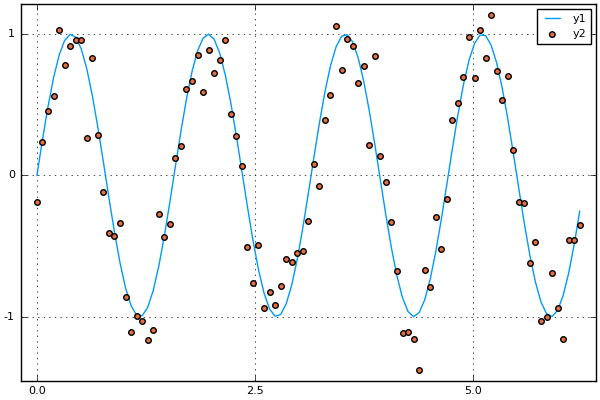To do this we will use cubic splines. The spline basis with knots at $\xi_i$ is given by $(x-\xi_i)^3_i$ . This can be expressed in terms of so-called B-splines by the formula,

$$p(x) = \sum_i c_i B_{i,3}(x).$$

The B-splines are a nice basis for the space of cubic splines, because they are smooth at the knots $\xi_i$. They are defined recursively in the following fashion:

$$B_{i,0}(x):=\left\{\begin{matrix}1&\mathrm {if} \quad t_{i}\leq x < t_{i+1}\\0&\mathrm {otherwise} \end{matrix}\right\}$$
$$B_{i,k}(x):={\frac {x-t_{i}}{t_{i+k}-t_{i}}}B_{i,k-1}(x)+{\frac {t_{i+k+1}-x}{t_{i+k+1}-t_{i+1}}}B_{i+1,k-1}(x).$$

This formula, and the corresponding recursive formula for the derivatives of beta splines are implemented by the following pair of functions. B-splines are typically used because they have compact support, which can speed up evaluation. I do not take advantage of this here.

function B(i, k, knots)
e = eps(0.0)
L = knots[end] - knots
# pad the knots vector for periodic case
knots = [knots..., (L+knots[2:2+k]-knots)...]

if k == 0
x-> float( knots[i] - e .<= x .< knots[i+1] + e)
else
x->(B(i,k-1, knots)(x) .* (x- knots[i])/(knots[i+k]-knots[i])
+ B(i+1,k-1, knots)(x) .* (knots[i+k+1]-x)/(knots[i+k+1]-knots[i+1]))
end
end

function Bder(i, k, knots, p)
e = eps(0.0)
L = knots[end] - knots
# pad the knots vector for periodic case
knots = [knots..., (L+knots[2:2+k]-knots)...]

if p > k
x -> zero(x)
elseif p == 0
x -> B(i,k,knots)(x)
else
x->((Bder(i,k-1, knots,p)(x) .* (x- knots[i]) + Bder(i,k-1, knots,p-1)(x))/(knots[i+k]-knots[i])
+ (Bder(i+1,k-1, knots,p)(x) .* (knots[i+k+1]-x) - Bder(i+1,k-1,knots,p-1)(x))/(knots[i+k+1]-knots[i+1]))
end
end

Bder (generic function with 1 method)


This is what the B-splines for orders 0-3 look like.

knots = collect(0:.1:1.0)
xx = linspace(0,1,200)
plot(xx,[B(4, k, knots)(xx) for k in 0:3])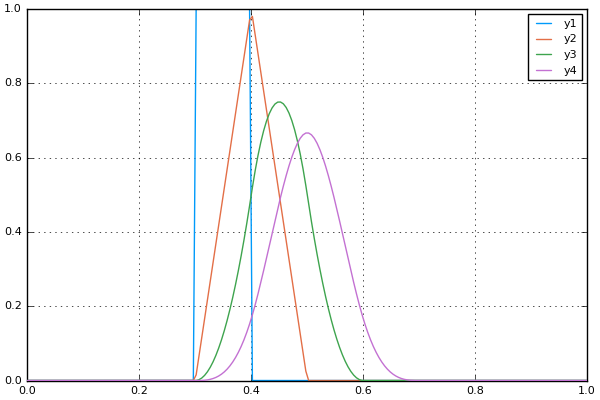And these are the first through third derivatives of the 3-rd order B-spline.

plot([Bder(4, 3, knots, p)(xx) for p in 0:2])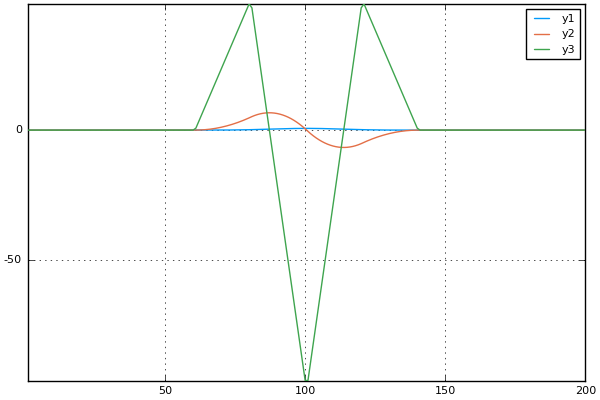## Smoothing using splines

Now I return to the original problem, and use a spline basis to smooth the noisy observations.

n = 20
p = 3
knots = collect(-p:n-1)*(2*pi)/n;

sp3basis = hcat([B(i,p,knots)(x) for i in 1:length(knots)]...);

yhat = sp3basis * (sp3basis \ yn);

plot(yhat)
plot!(y)
# scatter!(yn)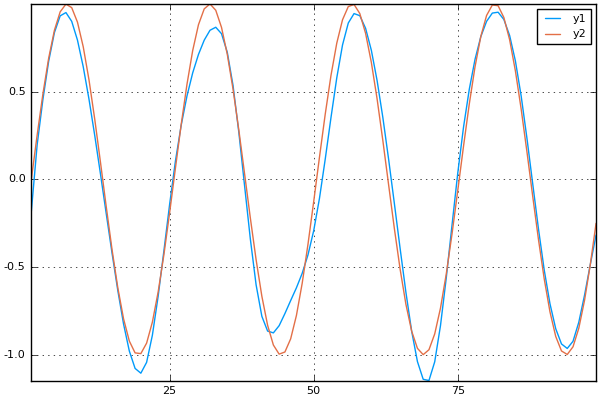While this looks okay, we are not taking advantage of the boundary conditions. For periodic boundaries, we demand that the first and second derivatives be continuous at the boundary.

$$\sum c_i B_{i,3}^{(p)}(0) = \sum c_i B_{i,3}^{(p)}(L),\ \text{for p = 0,1,2}$$
periodic_constraints = [Bder(i,3,knots, p)(x) - Bder(i,3,knots, p)(x[end]) for p=0:2, i=1:length(knots)]

3×23 Array{Float64,2}:
0.333333    1.33333   0.333333  0.0  …  -0.558076   -0.164105  -0.00423442
-3.1831      0.0       3.1831    0.0     -0.0909283  -0.674302  -0.0506727
10.1321    -20.2642   10.1321    0.0      1.42003    -0.40682   -0.202131


This is a projection operator which applies the constraints.

$y = A (I - B B^{\dagger}) c + \epsilon$

P = eye(size(periodic_constraints,2)) - pinv(periodic_constraints)*periodic_constraints;


Indeed the constraints are satisfied for random vectors of cofficents

periodic_constraints*P * rand(n+3)

3-element Array{Float64,1}:
1.70889e-15
1.08728e-15
-2.96689e-15


Let’s perform a least squares fit

A = sp3basis * P

beta = A\yn

yhat = A* beta
yhat = yhat;


The spline does a decent job at fitting the data.

plot(yhat)
plot!(y)
scatter!(yn)